# Ratios Proportions and the Geometric Mean Ratios A

• Slides: 18Ratios, Proportions, and the Geometric Mean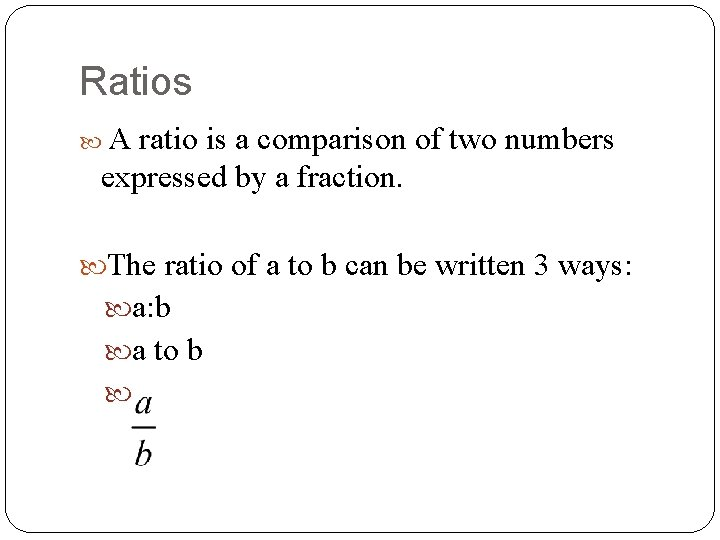Ratios A ratio is a comparison of two numbers expressed by a fraction. The ratio of a to b can be written 3 ways: a: b a to b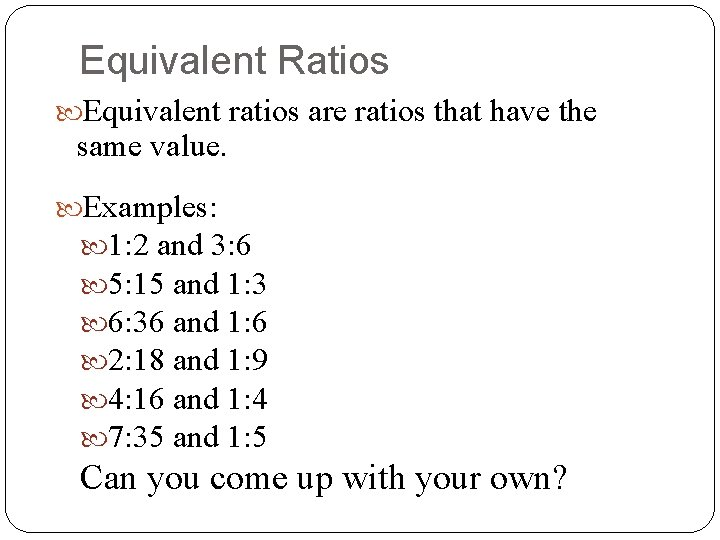Equivalent Ratios Equivalent ratios are ratios that have the same value. Examples: 1: 2 and 3: 6 5: 15 and 1: 3 6: 36 and 1: 6 2: 18 and 1: 9 4: 16 and 1: 4 7: 35 and 1: 5 Can you come up with your own?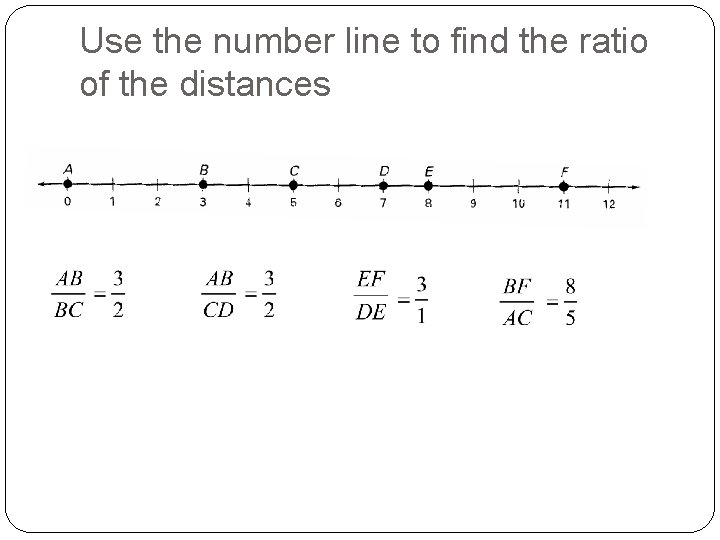Use the number line to find the ratio of the distances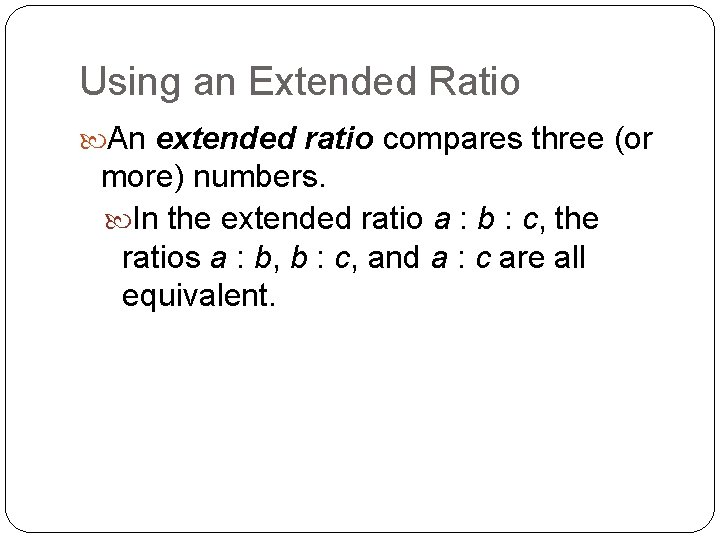Using an Extended Ratio An extended ratio compares three (or more) numbers. In the extended ratio a : b : c, the ratios a : b, b : c, and a : c are all equivalent.The lengths of the sides of a triangle are in the extended ratio 4 : 7 : 9. The perimeter is 60 cm. What are the lengths of the sides?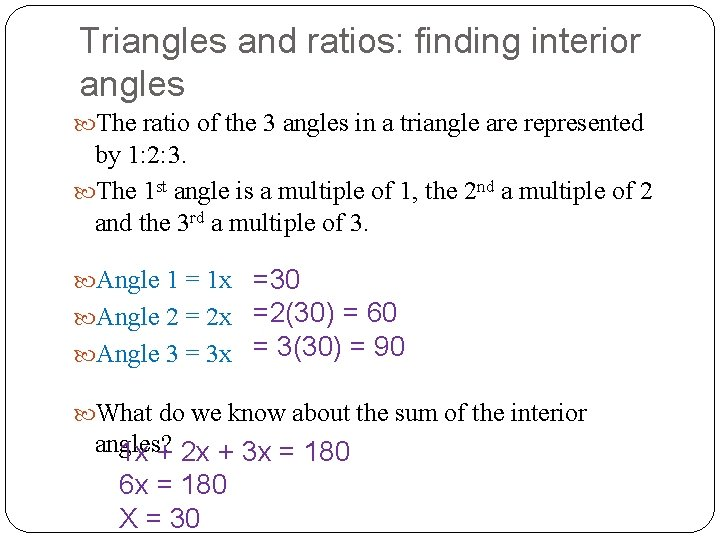Triangles and ratios: finding interior angles The ratio of the 3 angles in a triangle are represented by 1: 2: 3. The 1 st angle is a multiple of 1, the 2 nd a multiple of 2 and the 3 rd a multiple of 3. =30 Angle 2 = 2 x =2(30) = 60 Angle 3 = 3 x = 3(30) = 90 Angle 1 = 1 x What do we know about the sum of the interior angles? 1 x + 2 x + 3 x = 180 6 x = 180 X = 30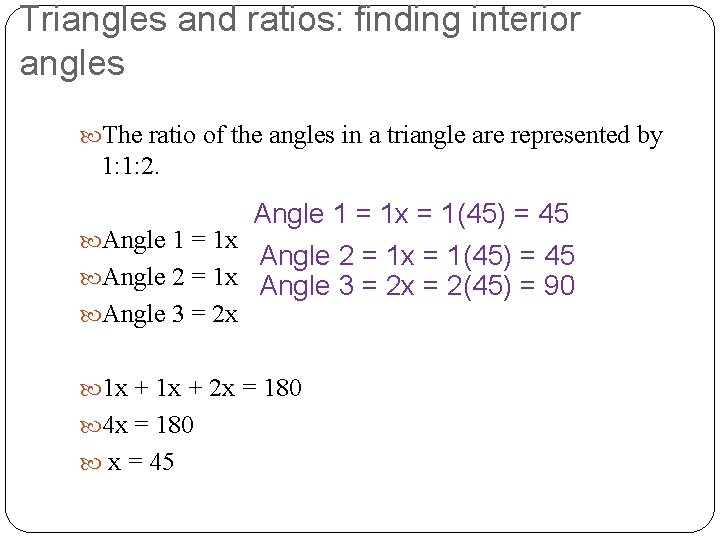Triangles and ratios: finding interior angles The ratio of the angles in a triangle are represented by 1: 1: 2. Angle 1 = 1 x = 1(45) = 45 Angle 1 = 1 x Angle 2 = 1 x = 1(45) = 45 Angle 2 = 1 x Angle 3 = 2 x = 2(45) = 90 Angle 3 = 2 x 1 x + 2 x = 180 4 x = 180 x = 45Proportions, extremes, means Proportion: a mathematical statement that states that 2 ratios are equal to each other. mea ns extremes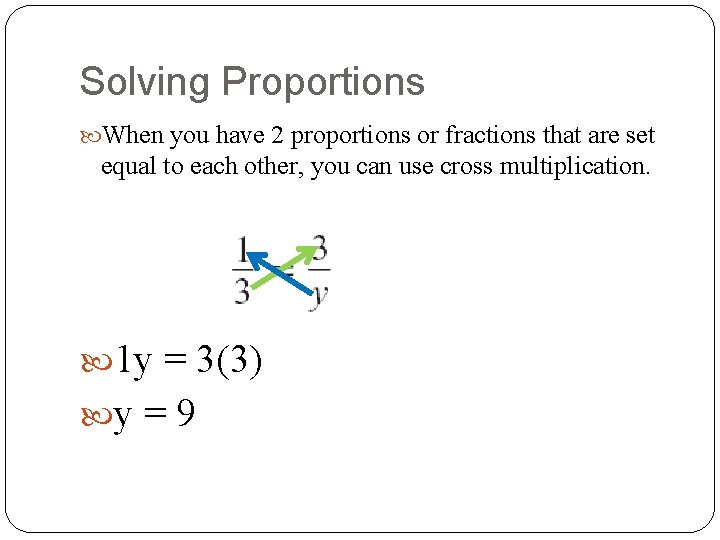Solving Proportions When you have 2 proportions or fractions that are set equal to each other, you can use cross multiplication. 1 y = 3(3) y = 9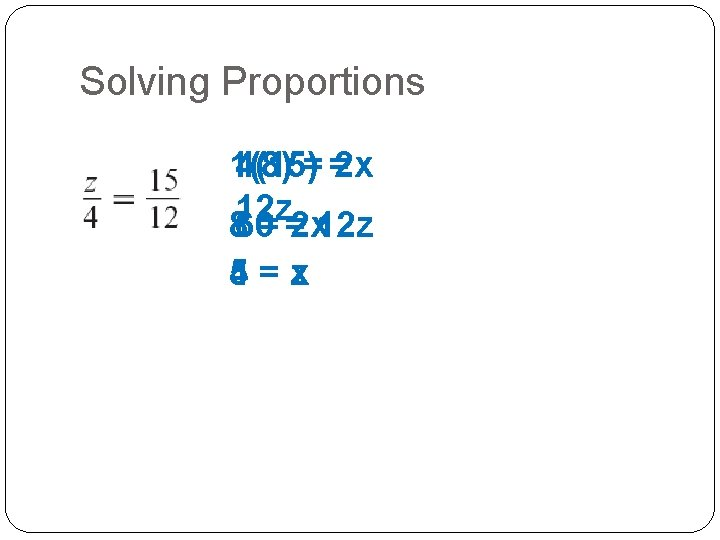Solving Proportions 1(8) 4(15)= =2 x 12 z 860= =2 x 12 z 4 = zx 5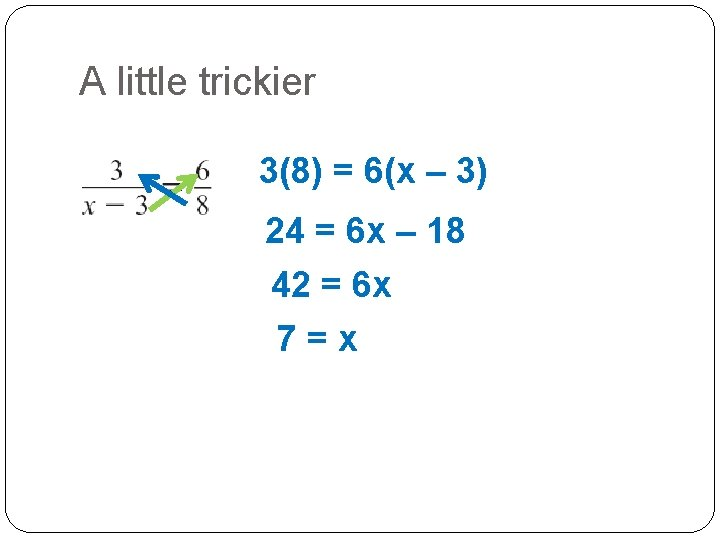A little trickier 3(8) = 6(x – 3) 24 = 6 x – 18 42 = 6 x 7=xX’s on both sides? 3(x + 8) = 6 x 3 x + 24 = 6 x 24 = 3 x 8=xNow you try!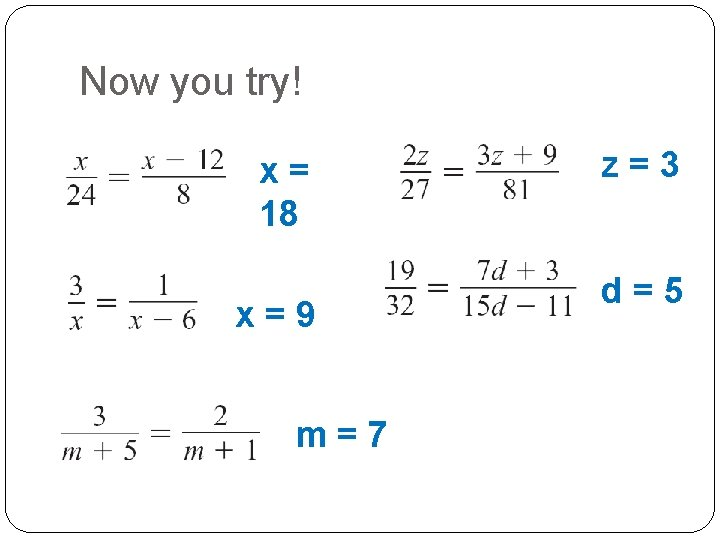Now you try! x= 18 x=9 m=7 z=3 d=5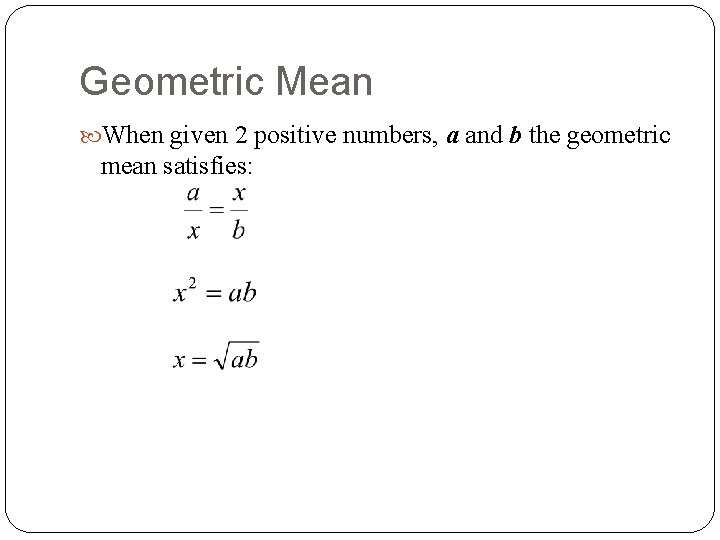Geometric Mean When given 2 positive numbers, a and b the geometric mean satisfies:Find the geometric mean x=2 x=3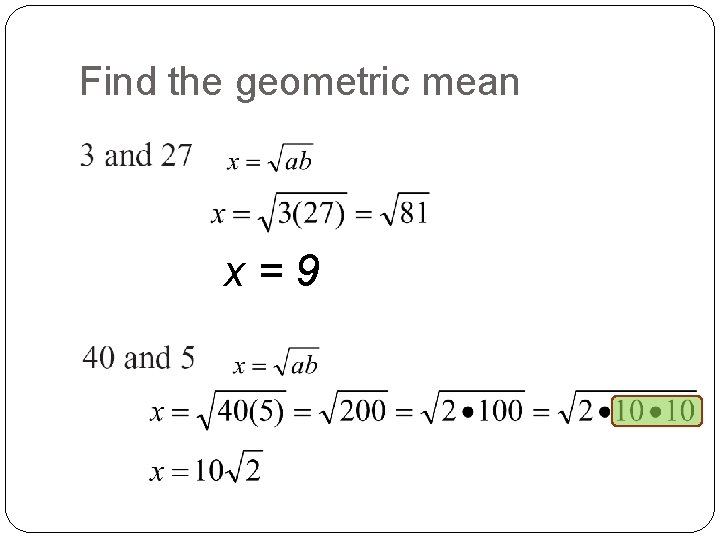Find the geometric mean x=9